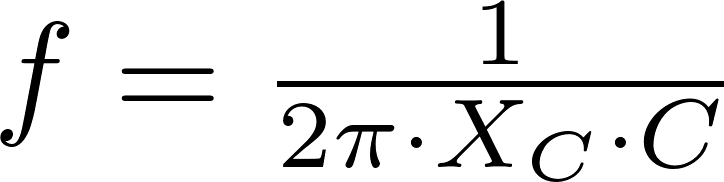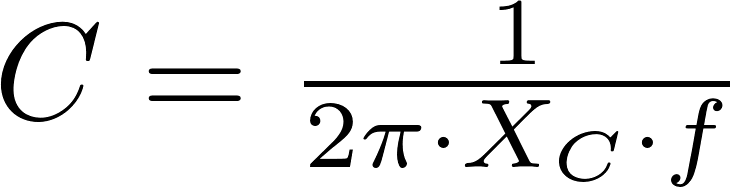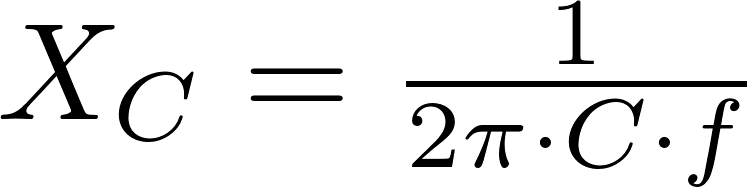# Capacitive reactance

Capacitive reactance is the opposition to the change of voltage across an element. The reactance gets larger if the frequency and capacitance get smaller.

### Formulas

The reactance can be calculated with the formula below. The reactance is positive in these formulas, so you have to add a negative sign when you want to calculate the impedance.f is the symbol for frequency and is measured in hertz (Hz).
C is the symbol for capacitance and is measured in farad (F).
X C is the symbol for capacitive reactance and is measured in (Ω).